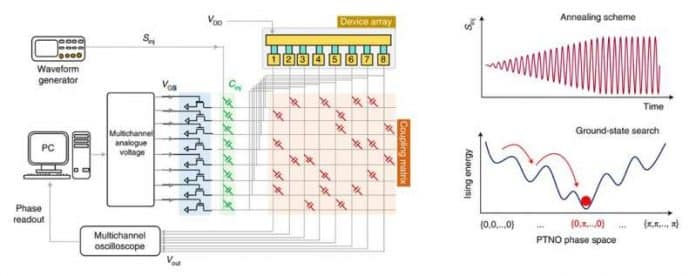# Complex Computational Problems Solver Based On Phase-Transition Nano-Oscillators

190Researchers developed a novel hardware that can solve combinatorial optimization problems such as the Ising Hamiltonian model more efficiently than existing devices.

Combinatorial optimization problems are a class of hard computational problems, involving finding an optimal object out of a finite set of objects. These combinatorial optimization algorithms are capable of processing huge amounts of data to predict or recommend an event or object based on past events and activities.

As the complexity of such computational problems grow, greater computational power and resources will be required to solve it. Researchers across the globe are trying to develop devices and platforms that could solve this type of computational problems faster and more efficiently, ranging from optical to electronic and quantum techniques.

Contributing to this trend, researchers at University of Notre Dame, Georgia Institute of Technology and Cornell University have developed an Ising Hamiltonian solver that may solve combinatorial optimization problems more efficiently than existing devices. The solver is based on coupled stochastic phase-transition nano-oscillators (PTNOs), that work similarly as spiking neurons in the brain.

The Ising Hamiltonian is a mathematical model of ferromagnetism in statistical mechanics. According to the researchers, the computational power is due to the dense connectivity between the computing elements. They describe that the massively parallel network is well suited for solving combinatorial optimization problems.

Based on this, the researchers developed a hardware of coupled PTNOs that can perform massively parallel searches through nearly all possible candidates and return the correct solution. The energy dissipation, according to the researchers, is is more than 10,000x lower than using our desktop computers.

“Through experimental demonstration and rigorous mathematical treatment, this work lays a foundation for utilizing the continuous-time dynamics of an interacting network of coupled non-linear oscillators to perform parallel computation and solve mathematically challenging combinatorial optimization problems,” Sourav Dutta, a post-doctoral researcher involved in the study, said. “From a practical implementation perspective, the key figure-of-merits for an Ising solver include the size of the hardware, operating temperature and the time and energy spent in obtaining the solution of the optimization problem.”

The research appeared in the journal Nature Electronics.